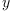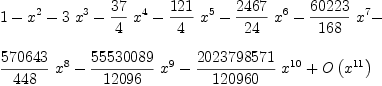login  home  contents  what's new  discussion  bug reports help  links  subscribe  changes  refresh  edit

 Submitted by : (unknown) at: 2007-11-17T22:21:41-08:00 (15 years ago) Name : Axiom Version : default friCAS-20090114 Axiom-20050901 OpenAxiom-20091012 OpenAxiom-20110220 OpenAxiom-Release-141 Category : Axiom Aldor Interface Axiom Compiler Axiom Library Axiom Interpreter Axiom Documentation Axiom User Interface building Axiom from source lisp system MathAction Doyen CD Reduce Axiom on Windows Axiom on Linux Severity : critical serious normal minor wishlist Status : open closed rejected not reproducible fix proposed fixed somewhere duplicate need more info Optional subject :   Optional comment :

For some reason

axiom
y:=operator 'y(1)
Type: BasicOperator
axiom
seriesSolve(-1+(1-2*x)*y(x)-(1-4*x)*D(y(x),x)=0, y, x = 0, )
axiom
Compiling function %B with type List(UnivariateTaylorSeries(
Expression(Integer),x,0)) -> UnivariateTaylorSeries(Expression(
Integer),x,0)(2)
Type: UnivariateTaylorSeries(Expression(Integer),x,0)

fails. We need to expose UnivariateTaylorSeriesODESolver first:

axiom
)expose UnivariateTaylorSeriesODESolver
UnivariateTaylorSeriesODESolver is now explicitly exposed in frame
initial
seriesSolve(-1+(1-2*x)*y(x)-(1-4*x)*D(y(x),x)=0, y, x = 0, )
axiom
Compiling function %D with type List(UnivariateTaylorSeries(
Expression(Integer),x,0)) -> UnivariateTaylorSeries(Expression(
Integer),x,0)(3)
Type: UnivariateTaylorSeries(Expression(Integer),x,0)

Furthermore, the documentation of seriesSolve is not quite clear to me. Why does:

  seriesSolve(-1+(1-2*x)*y(x)+x*(1-4*x)*D(y(x),x)=0, y, x = 0, )


fail - it should give the Catalan numbers.

Martin

noticed possible duplicate --kratt6, Wed, 13 Dec 2006 06:41:06 -0600 reply
This one is at least related, if not identical? to #301

duplicate of #301, which was fixed in FriCAS --kratt6, Tue, 13 Jan 2009 04:08:03 -0800 reply
Status: open => duplicate

 Subject:   Be Bold !! ( 14 subscribers )